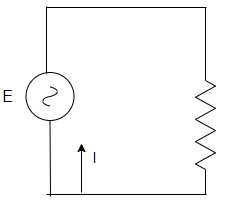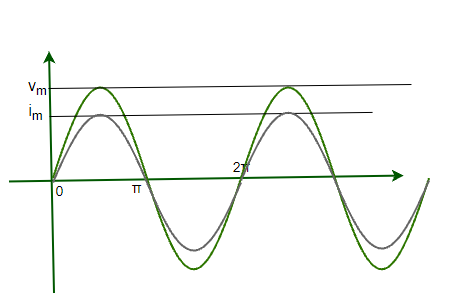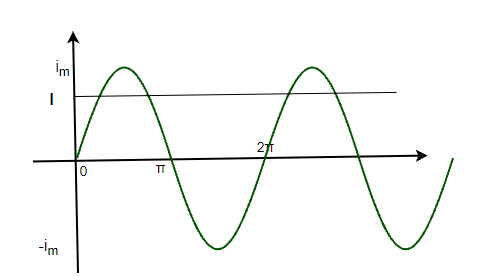GFG App
Open AppBrowser
Continue

# AC Voltage Applied to a Resistor

Alternating Currents are used almost as a standard by electricity distribution companies. In India, 50 Hz Alternating Current is used for domestic and industrial power supply. Many of our devices are in fact nothing but resistances. These resistances cause some voltage drop but since the voltage this time is alternating, these voltage drops are dealt with differently. It becomes essential to study the behavior when AC voltage is applied to a resistor.

### Alternating Current applied to a Resistor

In the figure given below, an alternating voltage source is shown. It is being applied to a resistor that is connected in series with the source. The source produces a sinusoidal varying potential difference across its terminals. Let us assume that this potential difference is called AC voltage. Then, this sinusoidally varying av voltage can be expressed by the equation given below,

v = vmsinωt

Here, vm is the amplitude of the oscillating voltage and ω denotes its angular frequency.For calculating the value of the current Kirchhoff’s law can be used.

∑ε(t) = 0

Applying this law to the circuit shown above,

v + iR = 0

vmsinωt  + iR =0

i = -vm/Rsinωt

So, the amplitude of the current is given by,

i =This equation is in compliance with ohm’s law. This means ohm’s law works for both dc and ac voltages. The figure is given below plots both of the values on the graph. Notice that both current and voltage go to maximum and become zero at the same time. This means that they have zero phase difference.The current also varies sinusoidally like the voltage. It goes on the positive side and increases in magnitude, then again decreases back and changes its direction. It can be inferred from the figure that the average current is also zero in a single cycle is zero.

### Power Dissipation

Even though the average current through the cycle is zero, but that does not mean that average power dissipation through the cycle is also zero. Dissipation of electrical energy is there. It’s known that Joule’s heating is given by i2R and depends on i2. This term is always positive irrespective of the sign of “i”. Thus average dissipation cannot become zero.

p = i2

⇒ p = (imsinωt)2R

⇒ p = (im)2sin2ωtR

This is the instantaneous power in the circuit. Average power dissipation is given by,

pavg = 1/2(im)2R = (vm)2/2R

Expressing this expression of power similar to the usual expression.

pavg = I2R = V2/R

WhereandThis is called the RMS value of the alternating current. For simplicity also, in our households voltages are specified by their RMS value. For example – the 230 V rating is an RMS value, it’s peak value with 311 V.

### Sample Problems

Question 1: Find the expression for the current flowing in the circuit with a resistance of 10 ohms. The voltage source works on the expression given, v = 10sin(t)

Using Kirchhoff’s law

∑ε(t) = 0

Applying this law to the circuit shown above,

v + iR = 0

10sin(t) + i(10) = 0

i = -sin(t)

Question 2: Find the expression for the current flowing in the circuit with a resistance of 50 ohms. The voltage source works on the expression given, v = 10sin(20t)

Using Kirchhoff’s law

∑ε(t) = 0

Applying this law to the circuit shown above,

v + iR = 0

10sin(20t) + i50 = 0

i = -0.2sin(20t)

Question 3: A circuit has two resistors of 20 and 30 ohms respectively. These resistors are connected in series. Find the expression for varying voltage across the 20-ohm resistor if the voltage source is given by, v = 10sin(20t)

Since these two resistors are in series, the equivalent resistance will be,

R = 20 + 30

⇒ R = 50

Using Kirchhoff’s law

∑ε(t) = 0

Applying this law to the circuit shown above,

v + iR = 0

10sin(20t) + i50 = 0

i = -0.2sin(20t)

The current through both resistors is same,

Voltage across the 20-ohm resistor is given by,

v = iR.

⇒ v = (-0.2sin(20t))(20)

⇒ v = -4sin(20t)

Question 4: A circuit has two resistors of 50 and 30 ohms respectively. These resistors are connected in series. Find the expression for varying voltage across the 30-ohm resistor if the voltage source is given by, v = 20sin(t)

Since these two resistors are in series, the equivalent resistance will be,

R = 50 + 30

⇒ R = 80

Using Kirchhoff’s law

∑ε(t) = 0

Applying this law to the circuit shown above,

v + iR = 0

20sin(t) + i(80) = 0

i = -0.25sin(t)

The current through both resistors is same,

Voltage across the 20-ohm resistor is given by,

v = iR.

⇒ v = (-0.25sin(t))(30)

⇒ v = -7.5sin(t)

Question 5: Average power dissipation in a circuit is given as 400W. The resistance of the circuit is 40 ohms. Find the peak value of the voltage in the circuit.

The Average power is given by,

P = V2/R

Given:

V = ?

R = 40

P = 400

plugging these values in the equation,

P = V2/R

⇒ 400 = (V)2 /40

⇒ V = √16000

V = 40√10 V

This is the rms value of the voltage.

Peak Value will be,

V = Vrms√2

⇒ V = 40√20

⇒ V = 80√5 V

My Personal Notes arrow_drop_up